# Tableau Charts & Graphs Tutorial: Types & ExamplesTableau can create interactive visualizations customized for the target audience. In this tutorial, you will learn about the measures, chart types and its features.

In this tutorial, ‘Sample-Superstore.csv’ is used for the demonstration. You can connect to the data source and follow the steps given in the tutorial

• Measure names and Measure values
• Generated Fields
• Understand how and when to build different types of Visuals
• Pareto Chart
• Bullet Chart
• Bar Chart
• Line chart
• Text Tables
• Heat Map
• Waterfall Chart
• Gantt chart
• Pie Chart
• Scatter Plot
• Area Chart
• Dual Axis Chart
• Bubble Chart
• Histogram

## Measure names and Measure values:

Measure names and Measure values are the two fields created in Tableau by default. These fields are created when a data set is imported into Tableau. You can go to a Data pane of the worksheet and view the fields as shown in the figure.Measure Names:

A measure name consists of all the names of the measure present in a data set. It always presents at the end of dimension list. It consists of discrete values of all measure names.

Measure Values:

All the measure values present in a data set are kept together in the field called measure values. It always presents at the end of measures list. It consists of continuous values of all measures.

Use cases of Measure Names and Measure Values:

Case 1:

Measure names and Measure values can be used to see the aggregation of all measure present in a data set. These fields can be shown as different types of visualization in Tableau.

Steps:

1. Drag ‘Measure Names’ into Columns.
2. Drag ‘Measure Values’ into Rows.It creates a visual for all measures present in the data set. By default, Tableau creates a bar chart showing all the measure names and their values.Case 2:

Any measures can be removed from the visual by removing the measure from mark card. It can be removed by

1. Right-click on a measure name.
2. Click on ‘Remove’ option.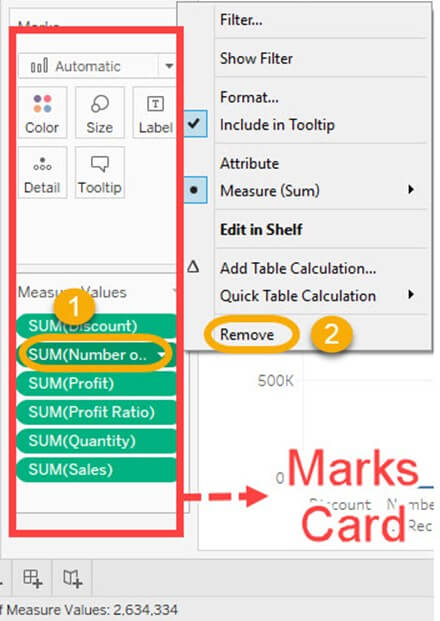It removes the measure from the visualization.Case 3:

Alias name can be created for measure names. It can be shown in the visualization for better identification.

Step 1) Use the same steps given in case 2 and follow the below steps.

1. Right click on measure name present in Columns.
2. Select the ‘Edit Alias’ option in the list.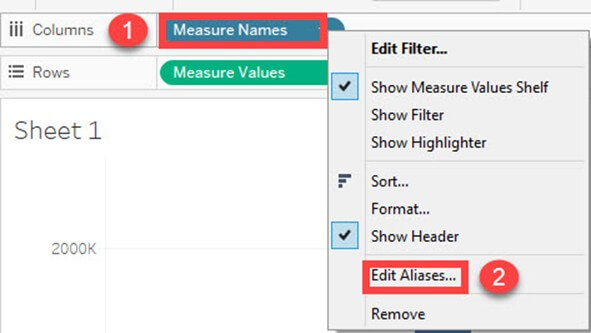Step 2) It opens ‘Edit Aliases’ Window.

1. Edit the alias name for the member. In this example, ‘Volume Sales’ is given as alias name for ‘Quantity’.
2. Click on OK.You can see the name change in Visualization.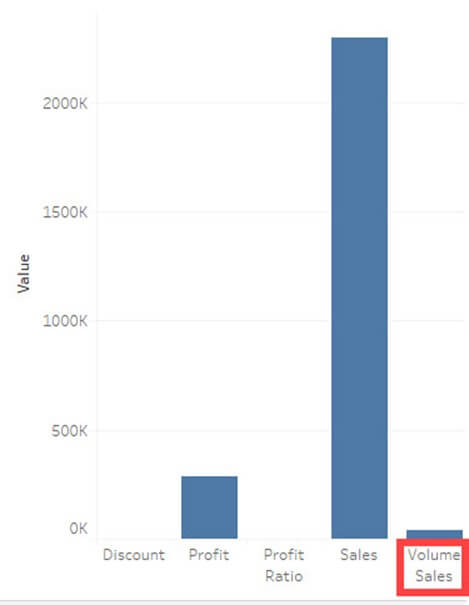Case 4:

On the off chance that you need to examine various measures in a solitary visual, it should be possible by utilizing measure names and measure esteems.

In this model, we will see the trend of measures specifically deals, profit and quantity over the years.

Stage 1)

• Drag measure esteems into the filter box.
• It opens a filter window.
• Look at all the measures aside from Profit, Quantity, and Sales.
• Snap on OK button.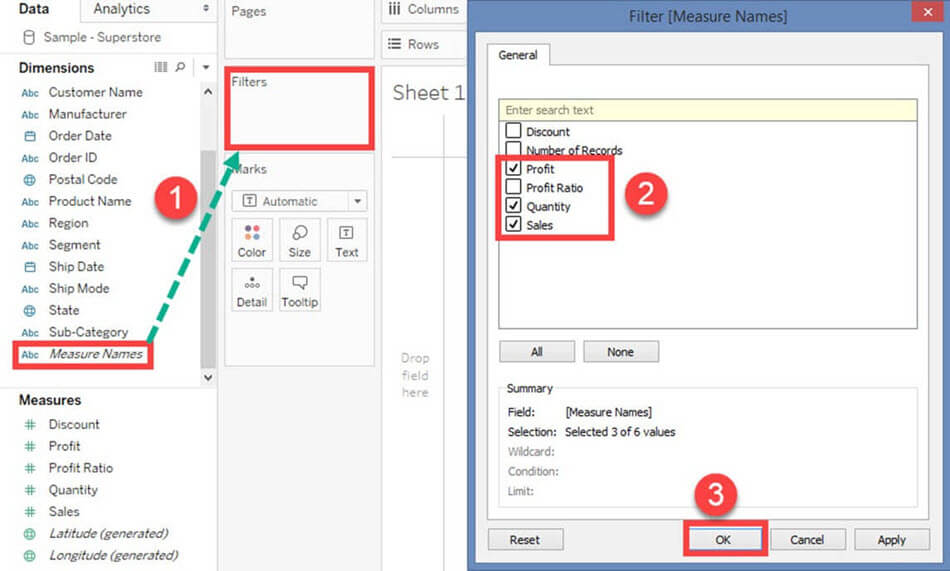It adds the measure name filter in the filter box.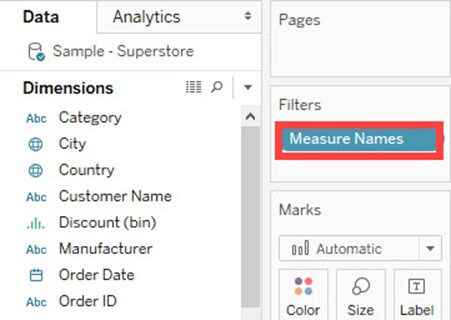For example: The above filter condition shows the visual as follows:It creates a filter on selected measures in the worksheet.

Step 2)

1. Drag ‘Order Date’ into Columns.
2. Drag ‘Measure Values’ into Rows.Stage 3)

Drag Measure Names into ‘Color’ choice present in the marks card.

It creates color of the visual dependent on the measure name. It likewise indicates different color to different measure names present in the visual.

A line chart is generated for numerous measures over years as appeared in the figure.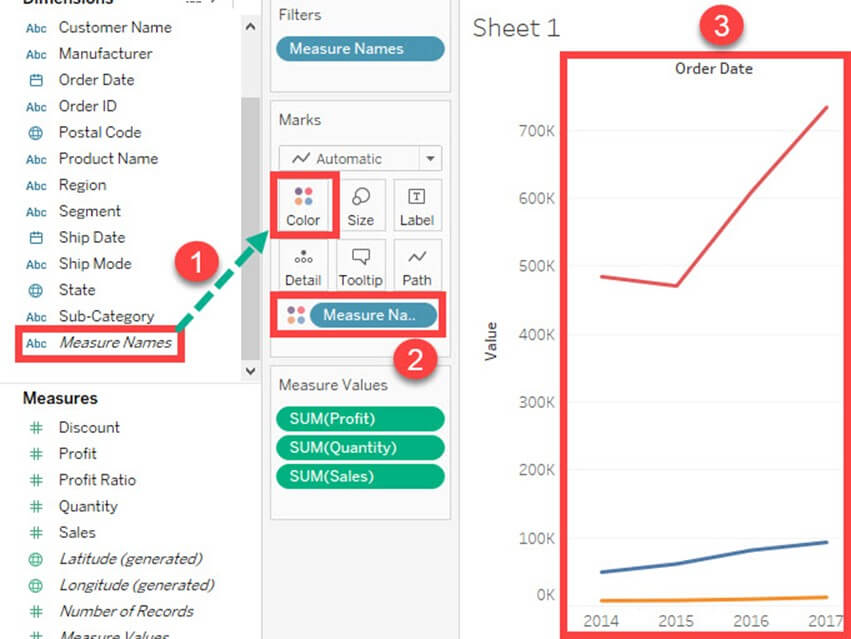## Generated Fields:

Tableau generates some fields which can be visible in the data pane. These fields are generated in addition to the fields present in the data set. The generated fields are given as follows.

• Measure Names
• Measure Values
• Number of Records
• Longitude
• Latitude

Measure Names and Measure Values are already explained in the article.

Number of Records:

Number of records shows the include of records present in the data set. It is an auto-generated field in Tableau which allots a worth ‘1’ for each record present in the data set. It can be utilized to verify the check of records when joining numerous tables. The procedure to check the number of records present in a dataset is given as follows.

Stage 1) Go to a Worksheet

Drag ‘Number of Records’ present in measure sheet into Rows.

It creates a bar chart as a matter of course. Hover over the bar to see the number of records present in the data set.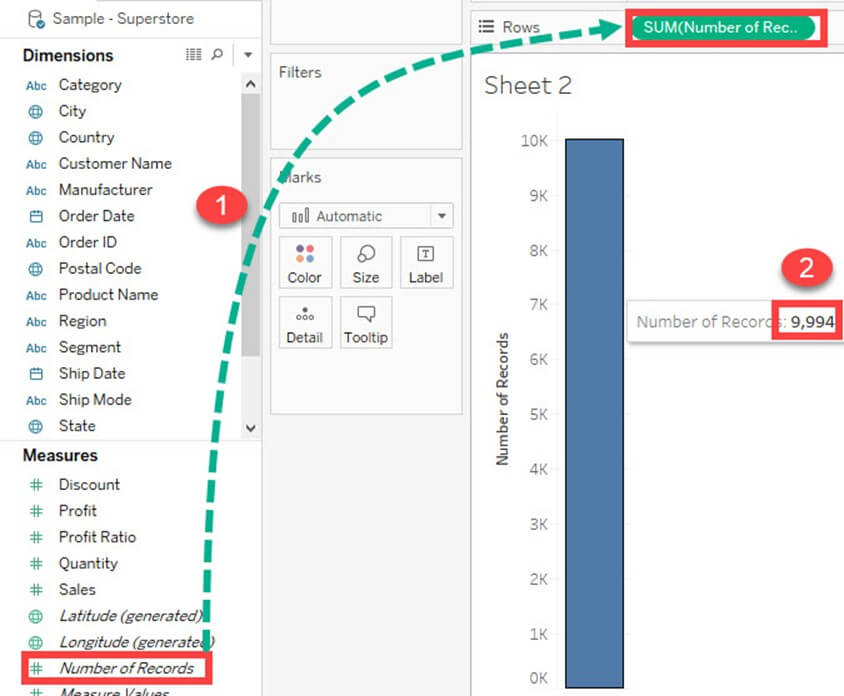Longitude and Latitude:

Longitude and Latitude (generated) fields are related with the geographical detail present in the data. Dataset should comprise of geographical subtleties like City, Country or State. The longitude and latitude esteems are auto-generated in Tableau. These fields can be utilized to construct maps in Tableau. You can create geographical guides utilizing Longitude and Latitude is given as follows.

Step 1) Drag Longitude (generated) into columns and Latitude (generated) into Rows.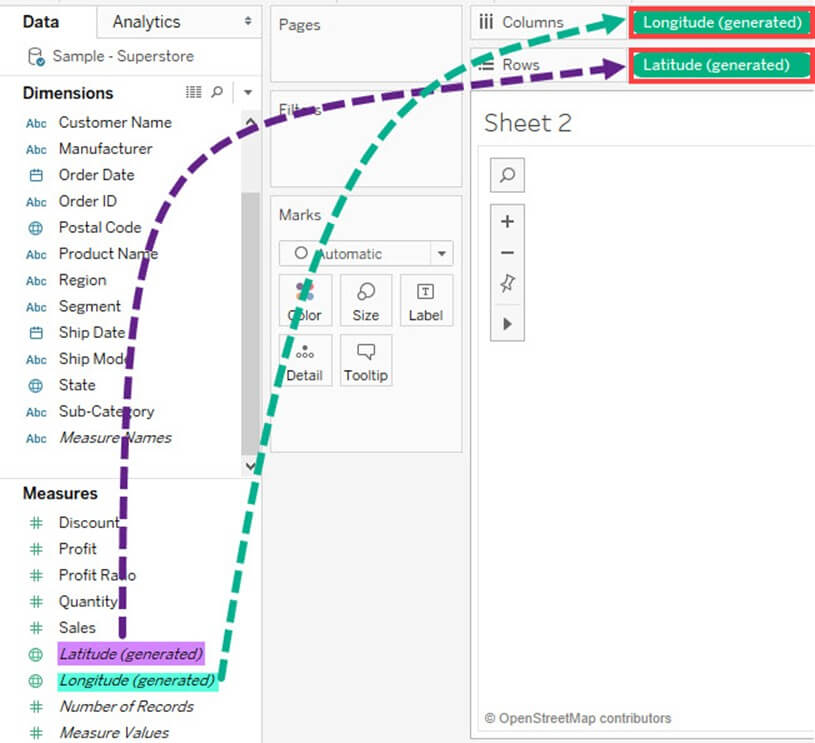Step 2) Drag ‘State’ from Dimension list into ‘Detail’ present in Marks Card.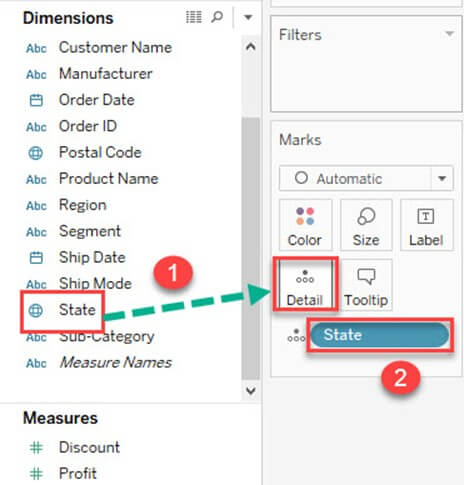### Pareto Chart:

A Pareto chart comprises of both bar and line graph. A similar measure is utilized to create the graphs however the measure esteems are controlled differently. The purpose of utilizing Pareto Chart in Tableau is to distinguish the contribution of members present in a field. For instance, Profit contributed by different subcategory of products in a retail store can be investigated utilizing Pareto Chart. It can show the top members and their contribution. The procedure to create a Pareto Chart is given as follows.

Step 1) Go to a new Worksheet

1. Drag ‘Sub-Category’ into Columns.
2. Drag ‘Profit’ into Rows.Step 2)

1. Right click on ‘Sub-Category’.
2. Select ‘Sort’ option from the list.Step 3) It opens a Sort Window.

1. Click on ‘Descending’ in Sort order.
2. Select ‘Field’ in ‘Sort by” section. Select the field as Profit and choose ‘Sum’ as aggregation.
3. Click on OK.Step 4)

1. Drag ‘Profit’ again into Rows.
2. Right-click on the newly added ‘Profit’ and Select ‘Dual axis’ option.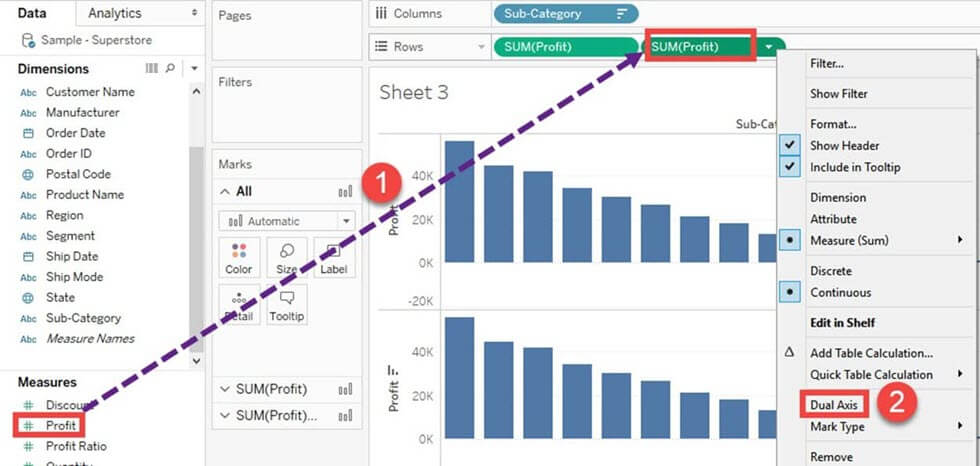It merges the x-axis of both measures and converts the visualization as given below.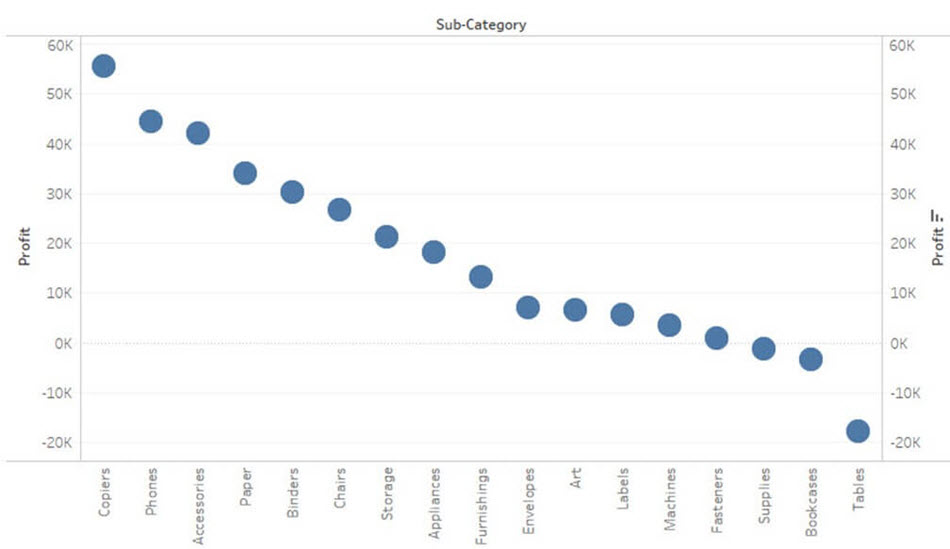Step 5) Go to Marks Card

1. Select ‘SUM(Profit)’ for the marks card list.
2. Click on the drop-down button as shown in the image.
3. Select ‘Bar’ as chart type.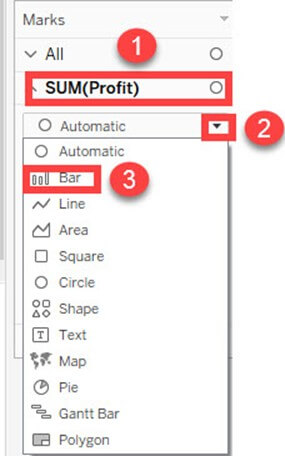Step 6)

1. Select ‘SUM(Profit)(2)’ from the marks card list.
2. Select the drop-down button as given in the image.
3. Click on ‘Line’ from the list.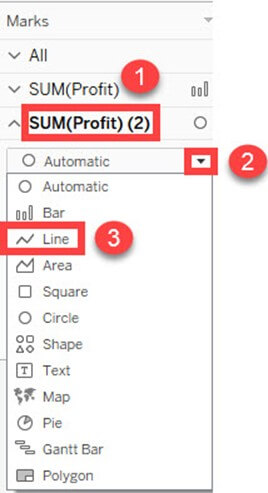Step 7)

1. Select ‘SUM(Profit)’ on the right side of rows as shown in the image.
2. Right click on it and select ‘Add Table Calculation’ from the list.Step 8) It opens the Primary Calculation Type window.

1. Select ‘Running Total’ from the drop-down.
2. Select ‘Sum’ as aggregation from the drop-down.
3. Click on Compute Using ‘Table (across)’.
4. Check in the ‘Add Secondary Calculation’ box.
5. It expands the window for ‘Secondary Calculation Type’. Select ‘Percent of Total’ from the dropdown list.
6. Select on Compute Using ‘Table (across)’
7. Now close the Window by clicking on close icon as shown in the image.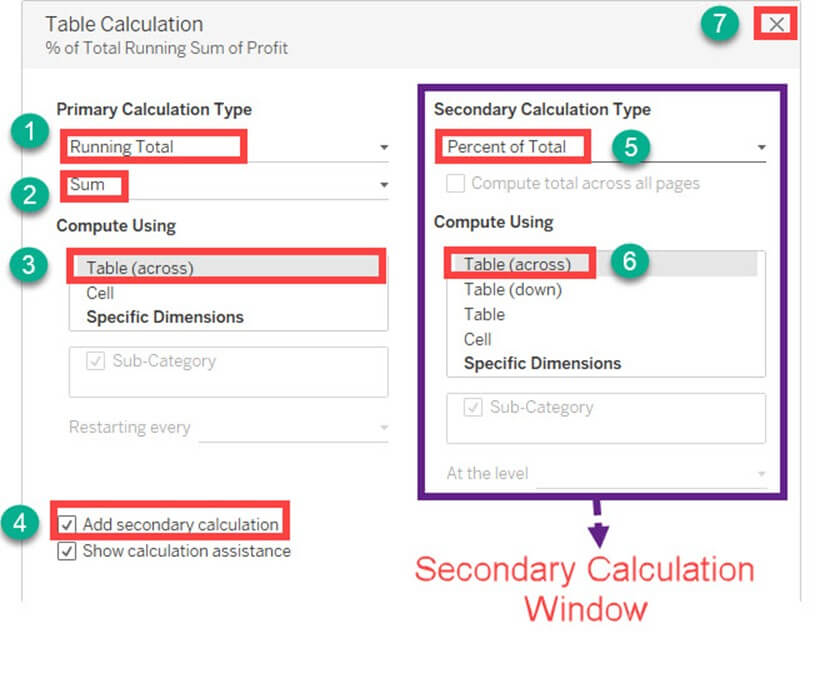Step 9)

1. Go to the last marks card namely ‘SUM (Profit)’.
2. Click on color icon present in the marks card.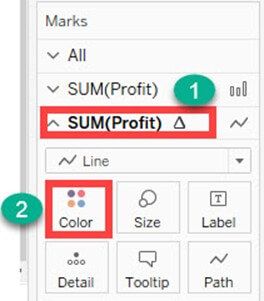Step 10) Select any color of your choice. This changes the color of line present in the graph.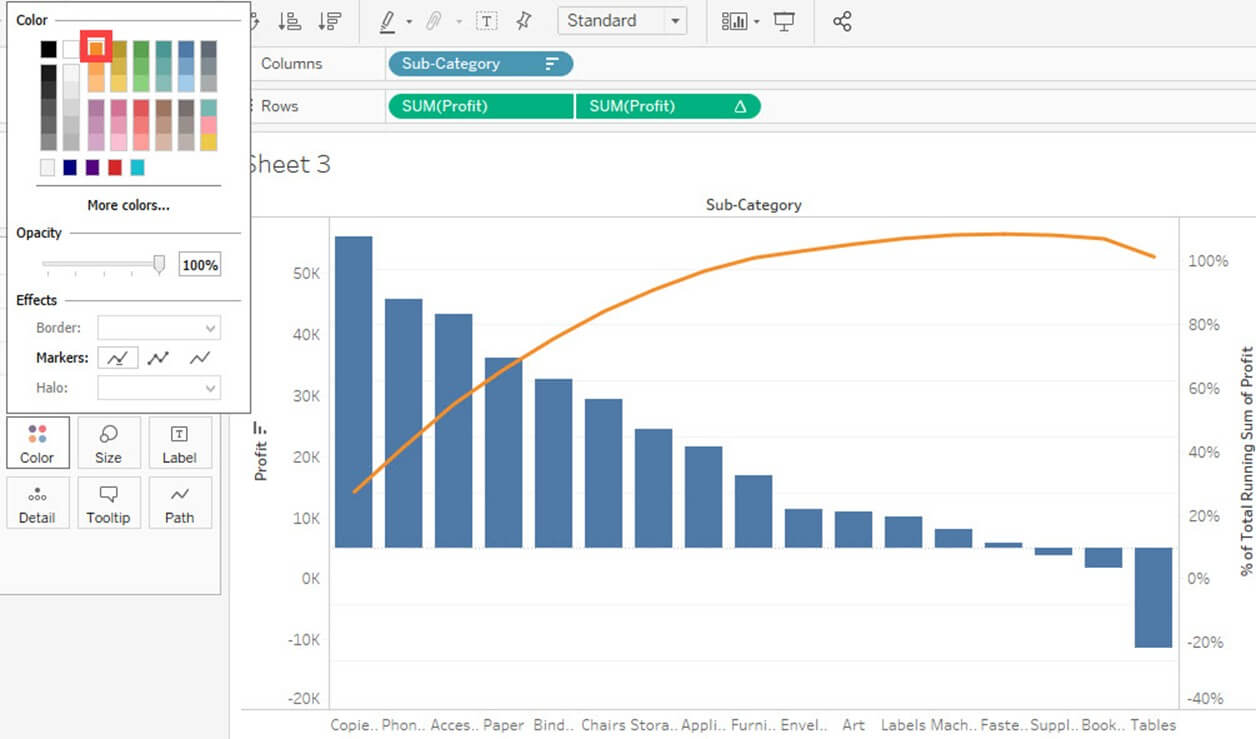### Bullet Chart:

A projectile chart can be utilized as a check or indicator to show the performance of measures. Two measures can be compared to one another utilizing the slug graph. For instance, on the off chance that we are having assessed profit and genuine profit we can compare them two utilizing projectile chart. The procedure to create slug chart is given as follows.

Stage 1) Go to another Worksheet.

1. Snap on ‘Examination’ present in the Menu bar.
2. Select ‘Create Calculated Field’ from the rundown.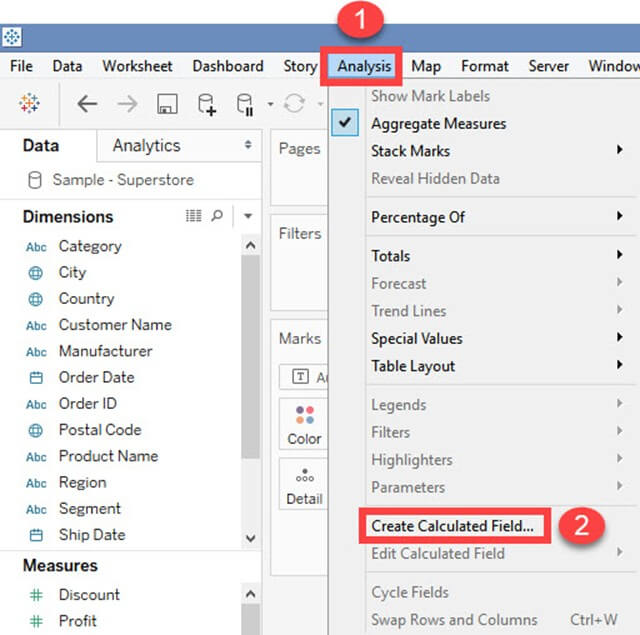Step 2) It opens Calculated Field Window.

1. Enter a name for the calculated field. In this example, it is named as ‘Estimated Profit’.
2. Type the estimated value of the measure. In this example, Profit is taken as the measure. So the calculated field is created for estimated profit.
3. Click on OK.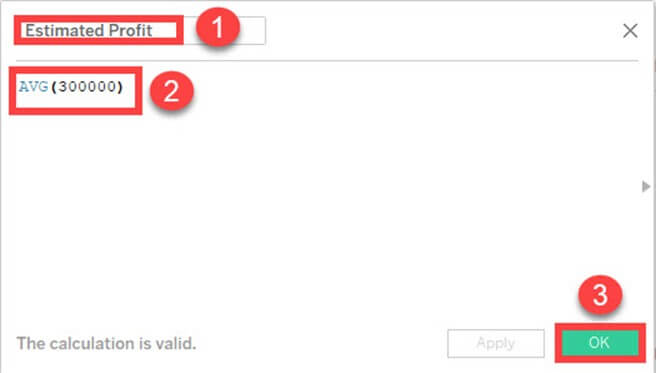Step 3) Go to measures in Data pane. Hold the control key in keyboard and select ‘Estimated Profit’ and ‘Profit’.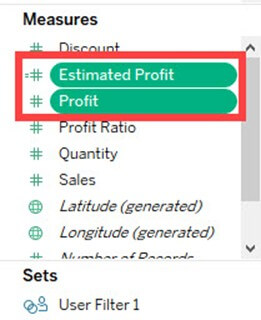Step 4)

1. Click on ‘Show me’ button present in the top right corner of the worksheet.
2. Select bullet graph icon as shown in the image.### Bar Chart:

A bar chart can compare the data across different categories. The stature of the bars represents the measured estimation of every category. It can be represented as vertical and horizontal sort bar charts. The procedure to create bar chart is given as follows.

Step) Go to another worksheet.

• Drag ‘Category’ into Column.
• Drag ‘Profit’ into Rows.
• It creates a bar chart naturally.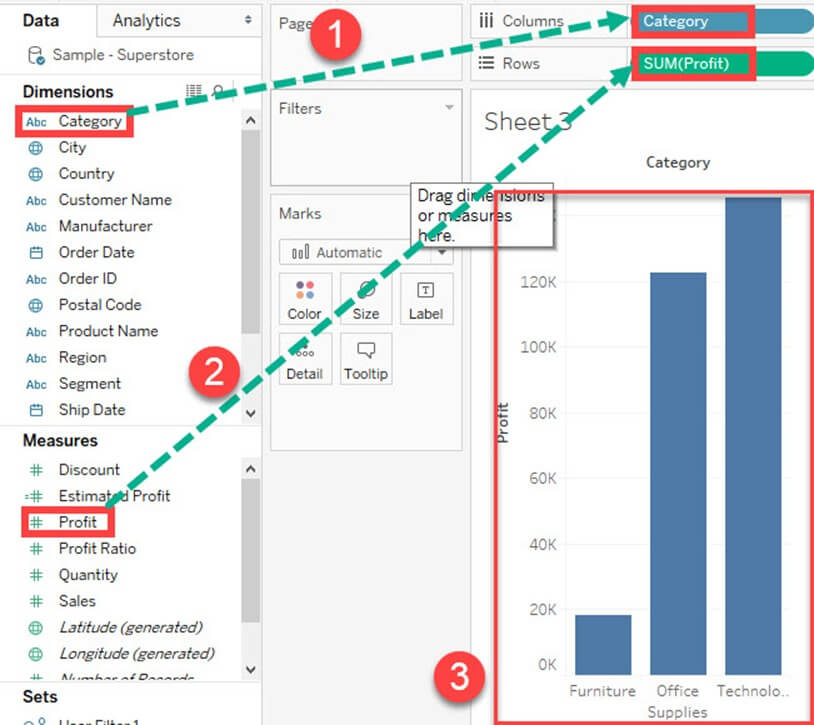### Line chart:

A Line Chart should be used to compare the data over the different periods. A line chart is created by the series of dots. These dots represent the measured value in each period. The procedure to create line graph is shown below.

Step) Go to a new Worksheet.

1. Drag ‘Order Date’ into Columns.
2. Drag ‘Sales’ into Rows.
3. It creates a line chart by default.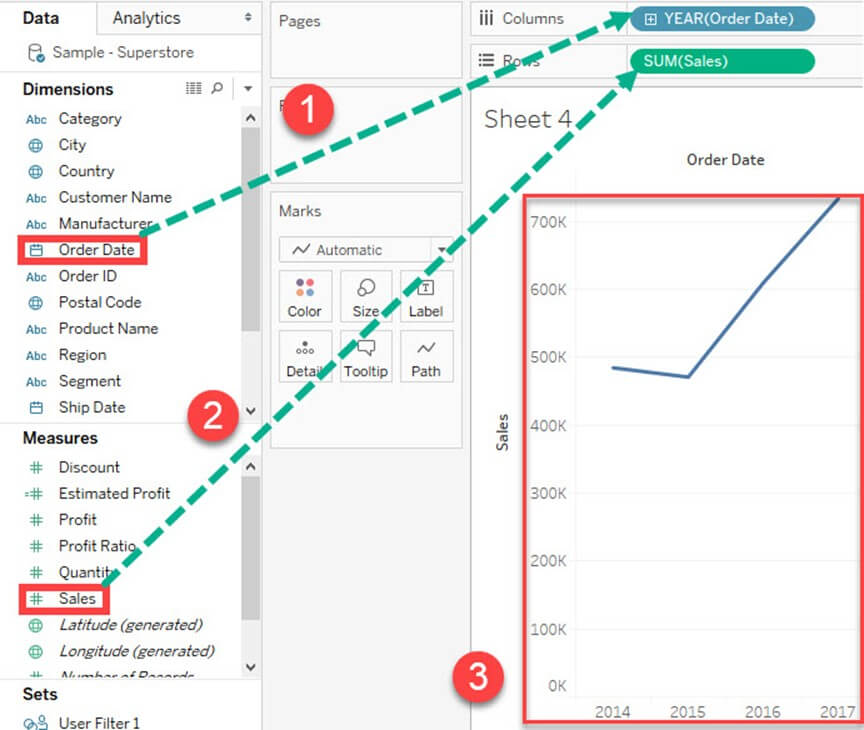Text Tables:

Text tables are utilized to show the specific estimation of measures across the different measurement. A book table is additionally called as a Pivot Table. It groups the measurements and measures of course. The procedure to structure a book table is given as follows.

Step) Go to another Worksheet.

• Drag ‘Sub-Category’ into Rows.
• Drag ‘Profit’ into text confine present the marks card.
• It creates a book table as a matter of course.